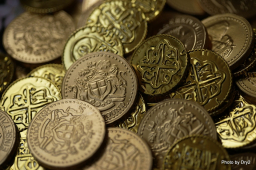# Treasury 73334

Peter has only two crowns in the treasury and can divide them both in a ratio of 4:5 and in a ratio of 4:7. How many crowns does Peter have in the money box if the amount saved is less than 300 CZK?

x1 =  198 Kc

### Step-by-step explanation:Did you find an error or inaccuracy? Feel free to write us. Thank you!

Tips for related online calculators
Do you want to calculate the least common multiple of two or more numbers?
Need help calculating sum, simplifying, or multiplying fractions? Try our fraction calculator.
Check out our ratio calculator.